Orners corner

Op-amp based Gain/Reference/Attenuation block

While working on verifying the dynamic range of an audio compressor project, I needed a faster method to switch between +10dBu, 0dBu and -10dBu without constantly adjusting the signal generator. What I needed was the ability to set the zero reference level, then go to the upper limit followed by the lower limit. There are many other possible applications where this can be used as well, such as managing levels between -10dBV consumer and +4dBu professional or vice-versa (i.e. +/-12db of gain/attenuation)

The basic form of a resistive voltage divider follows the equation: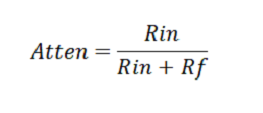Referring to the non-inverting amplifier configuration we can see that this resistive divider is connected from the output to the inverting input.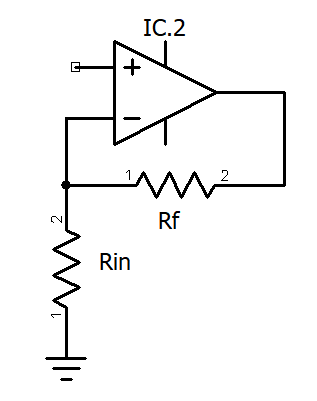Figure 1 - Non-Inverting Op-Amp

Therefore the gain equation of the non-inverting op-amp with feedback is the inverse of the resistive divider.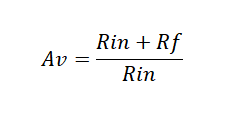A slight modification of the non-inverting op-amp by adding a 3-way switch in the feedback loop, provides three possible configurations. Reviewing the schematic in Fig 2 and the switch in Fig 3 will show that:

• It is connected as a voltage follower, driving the resistive attenuator, providing attenuation of x.
• It is connected as a voltage follower with the output taken from the inverting input, which tracks the non-inverting input, resulting in unity gain.
• It is connected as a negative feedback gain non-inverting op-amp with the output providing gain of x as the inverse of the attenuation x.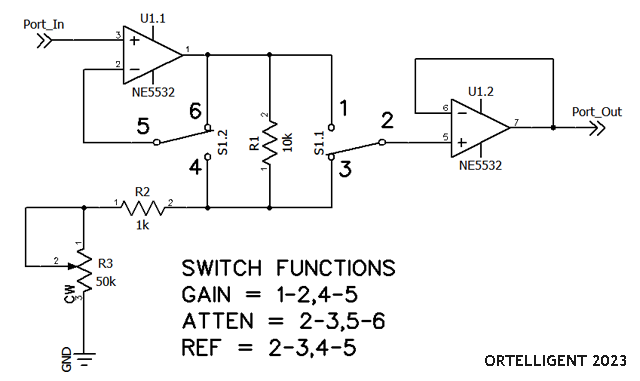Figure 2 - Gain/Ref/Atten Circuit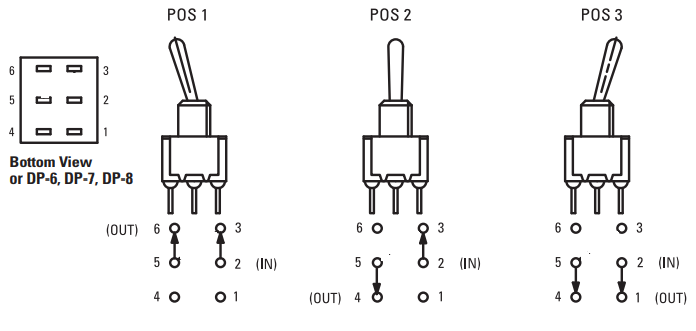Figure 3 - 3-way switch ( E-SWITCH 100DP6T1B1M2QE )

Being a non-inverting circuit, the minimum gain will be >1. The potentiometer can be replaced by a rotary switch with presets to select specific values of gain. The switch indicated is available from other sources in different form factors. This circuit simply forms the basis for implementation in other applications.